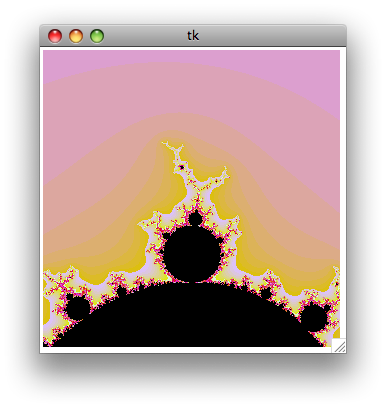# Mandelbrot evolution

20 mai 2008

As promised in my previous post, here is a more evolved version of my Python simple Mandelbrot generator. This version adds color and zoom support, but it remains limited — it is meant to be simple, plus I’m still a Python beginner.Read more to get the commented source :)

``````[python]import mathfrom Tkinter import *# Global variablescwidth = 300        # Width of the canvascheight = 300        # Height of the canvasloops = 200         # Maximum number of iterationszoomf = 4           # Zoom factorcomputing = False   # Wether we're being computing the fractal or not# Global mandelbrot window coordinatesmx1 = -2my1 = 2mx2 = 2my2 = -2# Create a simple (and ugly) color palettedef make_palette(loops):        # Create an empty array    palette = []        for i in range (0, loops):                # Generate an hex color code biased toward red        c = ((i * 1000) + 0xDD9900) % 0xFFFFFF                # Format the color code as #FFFFFF        color = "#%06X" % c        palette.append(color)            # The end of the palette must be black...    palette[loops-1] = "#000"    # ... and the start too    palette = "#000"    palette = "#000"        # Return the palette array    return palette# Mandelbrot function# cv : canvas# palette : the color palette array# x1, y1 : coordinates of the uper left point of the window# x2, y2 : coordinates of the bottom right point of the windowdef mandel(cv, palette, x1, y1, x2, y2):    # Clear the canvas    cv.delete(ALL)    # Compute the x and y increment    dx = float(abs(x2 - x1)) / cwidth    dy = float(abs(y2 - y1)) / cheight        # Let's start the maths    y = y1    for j in range(0, cheight):        x = x1        for i in range(0, cwidth):                        x = x + dx            c = complex(x, y)            a = 0            # Core loop : x = x^2 + c, loop n times, see if the number escape a circle centered on 0            for k in range(0, loops):                a = a*a + c                if abs(a) > 4:                    break                        # Draw a plot of a color from the palette,            # depending of when the point escaped from the loop            cv.create_line(i, j, i, j + 1, fill = palette[k])                    y = y - dy        cv.update()# Compute a rectangular zoom window from the zoom factor# cx, cy : coordinates of the center of the zoom window# unZoom : specifies if we're unzooming instead of the default zoomingdef computeZoomRect(cx, cy, unZoom = False):    global mx1, mx2, my1, my2, zoomf    if unZoom:        width = abs(mx2 - mx1) * float(zoomf)        height = abs(my2 - my1) * float(zoomf)    else:        width = abs(mx2 - mx1) / float(zoomf)        height = abs(my2 - my1) / float(zoomf)    mousex = (cx / float(cwidth)) * abs(mx2 - mx1) + mx1    mousey =  -(cy / float(cheight)) * abs(my2 - my1) + my1            zoomRect = [0, 0, 0, 0]    zoomRect = mousex - (width / float(2))    zoomRect = mousey + (height / float(2))    zoomRect = mousex + (width / float(2))    zoomRect = mousey - (height / float(2))    return zoomRect# Draw a preview of the zoom window on the canvasdef mouseMove(event):    global c, cwidth, cheight, zoomf, zoomRect, computing        # Draw rectangle only if we finished to compute the fractal    if computing == True:        return        # Compute rect half-width and half-height    hwidth = (cwidth / zoomf) / 2    hheight = (cheight / zoomf) / 2    # Delete previous zoom Rect    c.delete(zoomRect)        # Create a new zoomRect around the current mouse location    zoomRect = c.create_rectangle(event.x - hwidth, event.y - hheight, event.x + hwidth, event.y + hheight, width=1)        return# Zoom functiondef zoom(event):    global mx1, mx2, my1, my2, c, palette, computing        # If we are already computing, do nothing and return    if computing == True:        return        # Compute a zoom window    rect = computeZoomRect(event.x, event.y)        # Update the global coordinates    mx1 = rect    my1 = rect    mx2 = rect    my2 = rect        # Draw the fractal    computing = True    mandel(c, palette, mx1, my1, mx2, my2)    computing = False        return    # Unzoom functiondef unzoom(event):    global mx1, mx2, my1, my2, c, palette        # If we are already computing, do nothing and return    if computing == True:        return        # Compute a zoom window    rect = computeZoomRect(event.x, event.y, True)        # Update the global coordinates    mx1 = rect    my1 = rect    mx2 = rect    my2 = rect        # Draw the fractal    computing = True    mandel(c, palette, mx1, my1, mx2, my2)    computing = False        return# Create window and canvasroot = Tk()c = Canvas(root,width = cwidth, height = cheight)zoomRect = c.create_rectangle(0,0,0,0)c.pack()# Register eventsc.bind('', zoom)c.bind('', unzoom)c.bind('', mouseMove)# Create the palettepalette = make_palette(loops)# Draw first mandelbrotcomputing = Truemandel(c, palette, mx1, my1, mx2, my2)computing = False# Run event looproot.mainloop()
``````

Kudos

### Discussion, liens, et tweetsJ’écris des sites web, des logiciels, des applications mobiles. Vous me trouverez essentiellement sur ce blog, mais aussi sur Mastodon, parmi les Codeurs en Liberté, ou en haut d’une colline du nord-est de Paris.